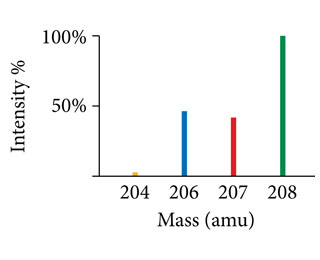Problem: Use the mass spectrum of lead to estimate the atomic mass of lead.

FREE Expert Solution
80% (410 ratings)
FREE Expert Solution

We are being asked to estimate the atomic mass of lead using the mass spectrum.

Recall: Mass Spectrometry allows you to determine the molecular weight of an unknown compound through its vaporization and ionization.

We are given the mass spectrum of lead with percent intensity on the vertical axis and mass (amu) on the horizontal axis.

The spectrum includes the percent intensities of four isotopes of lead:

• Pb-204 with a peak intensity of about 5.0%
• Pb-206 with a peak intensity of about 45%
• Pb-207 with a peak intensity of about 40%
• Pb-208 with a peak intensity of about 100%
80% (410 ratings)
Problem Details

Use the mass spectrum of lead to estimate the atomic mass of lead.What scientific concept do you need to know in order to solve this problem?

Our tutors have indicated that to solve this problem you will need to apply the Mass Spectrometry concept. If you need more Mass Spectrometry practice, you can also practice Mass Spectrometry practice problems .

How long does this problem take to solve?

Our expert Chemistry tutor, Jules took 3 minutes to solve this problem. You can follow their steps in the video explanation above.

What professor is this problem relevant for?

Based on our data, we think this problem is relevant for Professor Weiss' class at OREGONSTATE.

What textbook is this problem found in?

Our data indicates that this problem or a close variation was asked in . You can also practice practice problems .